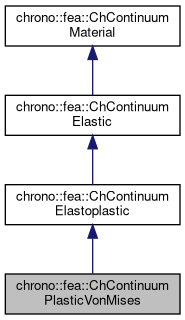chrono::fea::ChContinuumPlasticVonMises Class Reference

## Description

Class for the basic properties of materials in an elastoplastic continuum, with strain yield limit based on Von Mises yield.

#include <ChContinuumMaterial.h>

Inheritance diagram for chrono::fea::ChContinuumPlasticVonMises:[legend]
Collaboration diagram for chrono::fea::ChContinuumPlasticVonMises:[legend]

## Public Member Functions

ChContinuumPlasticVonMises (double myoung=10000000, double mpoisson=0.4, double mdensity=1000, double melastic_yeld=0.1, double mplastic_yeld=0.2)
Create a continuum isotropic elastoplastic material, where you can define also plastic and elastic max. More...

ChContinuumPlasticVonMises (const ChContinuumPlasticVonMises &other)

void Set_elastic_yeld (double melastic_yeld)
Set the elastic yield modulus as the maximum VonMises equivalent strain that can be withstood by material before starting plastic flow. More...

double Get_elastic_yeld () const
Get the elastic yield modulus.

void Set_plastic_yeld (double mplastic_yeld)
Set the plastic yield modulus as the maximum VonMises equivalent strain that can be withstood by material before fracture. More...

double Get_plastic_yeld () const
Get the plastic yield modulus.

virtual void Set_flow_rate (double mflow_rate) override
Set the plastic flow rate. More...

virtual double Get_flow_rate () const override
Set the plastic flow rate.

virtual double ComputeYeldFunction (const ChStressTensor<> &mstress) const override
Return a scalar value that is 0 on the yield surface, <0 inside (elastic), >0 outside (incompatible->plastic flow)

virtual void ComputeReturnMapping (ChStrainTensor<> &mplasticstrainflow, const ChStrainTensor<> &mincrementstrain, const ChStrainTensor<> &mlastelasticstrain, const ChStrainTensor<> &mlastplasticstrain) const override
Correct the strain-stress by enforcing that elastic stress must remain on the yield surface, computing a plastic flow to be added to plastic strain while integrating.

virtual void ComputePlasticStrainFlow (ChStrainTensor<> &mplasticstrainflow, const ChStrainTensor<> &mestrain) const override
Compute plastic strain flow (flow derivative dE_plast/dt) from strain, according to VonMises strain yield theory.

virtual void ArchiveOUT (ChArchiveOut &marchive) override

virtual void ArchiveIN (ChArchiveIn &marchive) overridePublic Member Functions inherited from chrono::fea::ChContinuumElastoplastic
ChContinuumElastoplastic (double myoung=10000000, double mpoisson=0.4, double mdensity=1000)Public Member Functions inherited from chrono::fea::ChContinuumElastic
ChContinuumElastic (double myoung=10000000, double mpoisson=0.4, double mdensity=1000)
Create a continuum isotropic hookean material. More...

ChContinuumElastic (const ChContinuumElastic &other)

void Set_E (double m_E)
Set the Young E elastic modulus, in Pa (N/m^2), as the ratio of the uniaxial stress over the uniaxial strain, for hookean materials. More...

double Get_E () const
Get the Young E elastic modulus, in Pa (N/m^2).

void Set_v (double m_v)
Set the Poisson v ratio, as v=-transverse_strain/axial_strain, so takes into account the 'squeezing' effect of materials that are pulled (so, if zero, when you push the two sizes of a cube, it won't inflate). More...

double Get_v () const
Get the Young v ratio, as v=-transverse_strain/axial_strain.

void Set_G (double m_G)
Set the shear modulus G, in Pa (N/m^2), as the ratio of shear stress to the shear strain. More...

double Get_G () const
Get the shear modulus G, in Pa (N/m^2)

double Get_l () const
Get Lamé first parameter (the second is shear modulus, so Get_G() )

double Get_BulkModulus () const
Get bulk modulus (increase of pressure for decrease of volume), in Pa.

double Get_WaveModulus () const
Get P-wave modulus (if V=speed of propagation of a P-wave, then (M/density)=V^2 )

void ComputeStressStrainMatrix ()
Computes Elasticity matrix and stores the value in this->StressStrainMatrix Note: is performed every time you change a material parameter.

ChMatrixDynamicGet_StressStrainMatrix ()
Get the Elasticity matrix.

void ComputeElasticStress (ChStressTensor<> &mstress, const ChStrainTensor<> &mstrain) const
Compute elastic stress from elastic strain (using column tensors, in Voight notation)

void ComputeElasticStrain (ChStrainTensor<> &mstrain, const ChStressTensor<> &mstress) const
Compute elastic strain from elastic stress (using column tensors, in Voight notation)

void Set_RayleighDampingM (double m_d)
Set the Rayleigh mass-proportional damping factor alpha, to build damping R as R=alpha*M + beta*K.

double Get_RayleighDampingM () const
Set the Rayleigh mass-proportional damping factor alpha, in R=alpha*M + beta*K.

void Set_RayleighDampingK (double m_d)
Set the Rayleigh stiffness-proportional damping factor beta, to build damping R as R=alpha*M + beta*K.

double Get_RayleighDampingK () const
Set the Rayleigh stiffness-proportional damping factor beta, in R=alpha*M + beta*K.Public Member Functions inherited from chrono::fea::ChContinuumMaterial
ChContinuumMaterial (double mdensity=1000)

ChContinuumMaterial (const ChContinuumMaterial &other)

void Set_density (double m_density)
Set the density of the material, in kg/m^2.

double Get_density () const
Get the density of the material, in kg/m^2.

## Additional Inherited MembersProtected Attributes inherited from chrono::fea::ChContinuumMaterial
double density

## ◆ ChContinuumPlasticVonMises()

 chrono::fea::ChContinuumPlasticVonMises::ChContinuumPlasticVonMises ( double myoung = 10000000, double mpoisson = 0.4, double mdensity = 1000, double melastic_yeld = 0.1, double mplastic_yeld = 0.2 )

Create a continuum isotropic elastoplastic material, where you can define also plastic and elastic max.

stress (yield limits for transition elastic->plastic and plastic->fracture).

## ◆ Set_elastic_yeld()

 void chrono::fea::ChContinuumPlasticVonMises::Set_elastic_yeld ( double melastic_yeld )
inline

Set the elastic yield modulus as the maximum VonMises equivalent strain that can be withstood by material before starting plastic flow.

It defines the transition elastic->plastic.

## ◆ Set_flow_rate()

 virtual void chrono::fea::ChContinuumPlasticVonMises::Set_flow_rate ( double mflow_rate )
inlineoverridevirtual

Set the plastic flow rate.

The lower the value, the slower the plastic flow during dynamic simulations.

Implements chrono::fea::ChContinuumElastoplastic.

## ◆ Set_plastic_yeld()

 void chrono::fea::ChContinuumPlasticVonMises::Set_plastic_yeld ( double mplastic_yeld )
inline

Set the plastic yield modulus as the maximum VonMises equivalent strain that can be withstood by material before fracture.

It defines the transition plastic->fracture.

The documentation for this class was generated from the following files:
• /builds/uwsbel/chrono/src/chrono/fea/ChContinuumMaterial.h
• /builds/uwsbel/chrono/src/chrono/fea/ChContinuumMaterial.cpp